# Support Vector Machines¶

Examples concerning the sklearn.svm module.Non-linear SVM

Non-linear SVM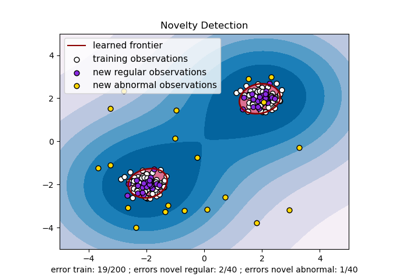One-class SVM with non-linear kernel (RBF)

One-class SVM with non-linear kernel (RBF)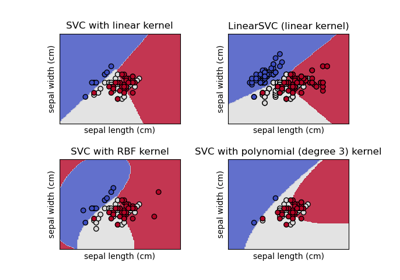Plot different SVM classifiers in the iris dataset

Plot different SVM classifiers in the iris dataset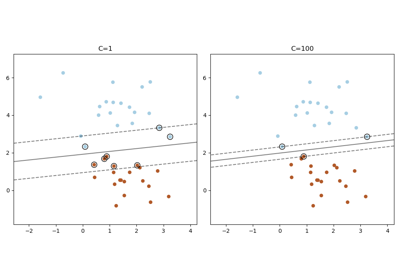Plot the support vectors in LinearSVC

Plot the support vectors in LinearSVC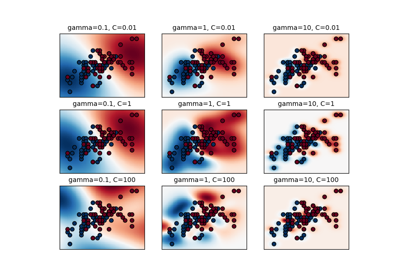RBF SVM parameters

RBF SVM parameters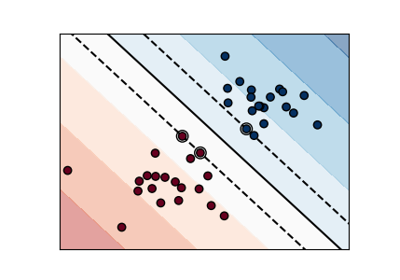SVM Margins Example

SVM Margins Example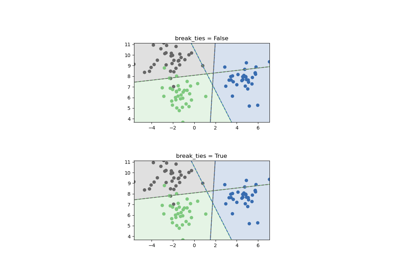SVM Tie Breaking Example

SVM Tie Breaking Example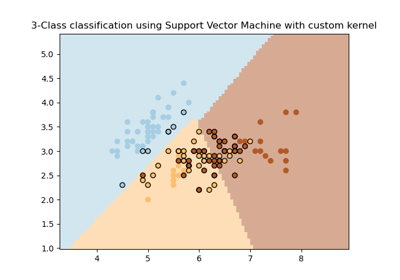SVM with custom kernel

SVM with custom kernel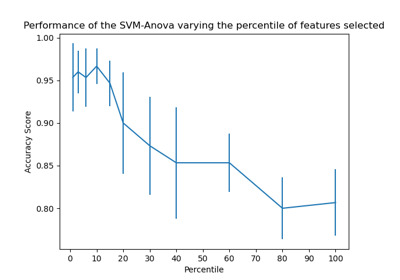SVM-Anova: SVM with univariate feature selection

SVM-Anova: SVM with univariate feature selection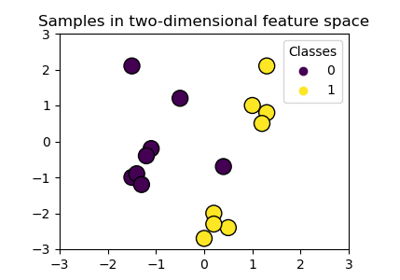SVM-Kernels

SVM-Kernels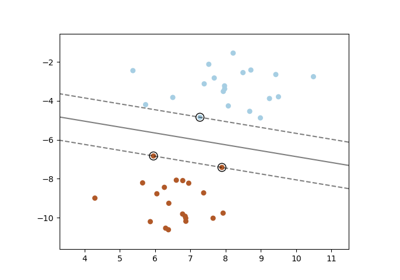SVM: Maximum margin separating hyperplane

SVM: Maximum margin separating hyperplane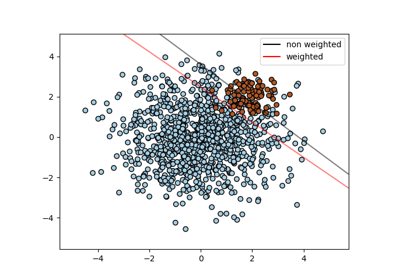SVM: Separating hyperplane for unbalanced classes

SVM: Separating hyperplane for unbalanced classes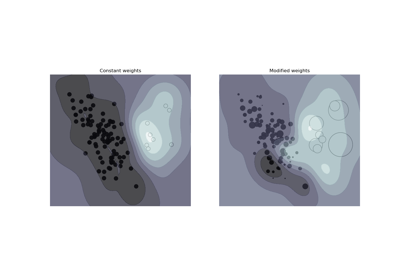SVM: Weighted samples

SVM: Weighted samples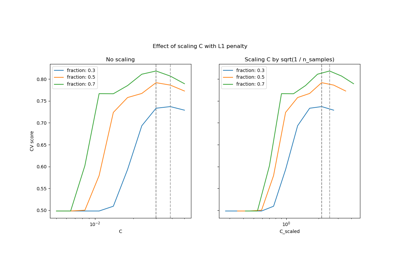Scaling the regularization parameter for SVCs

Scaling the regularization parameter for SVCs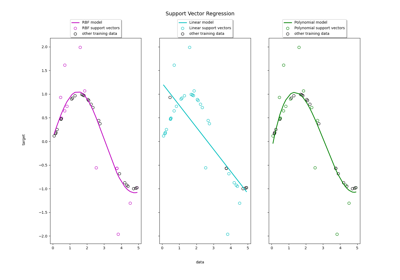Support Vector Regression (SVR) using linear and non-linear kernels

Support Vector Regression (SVR) using linear and non-linear kernels